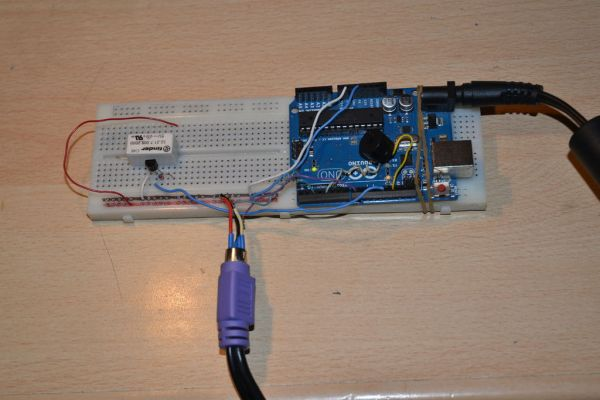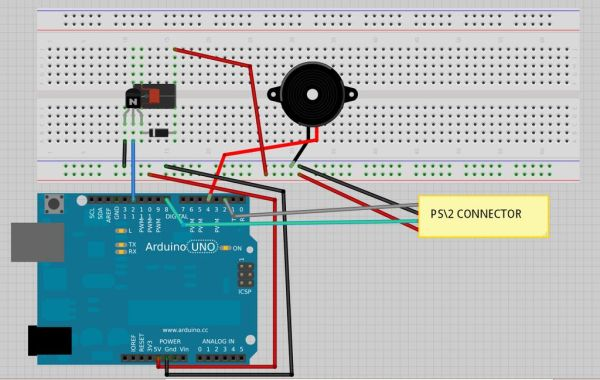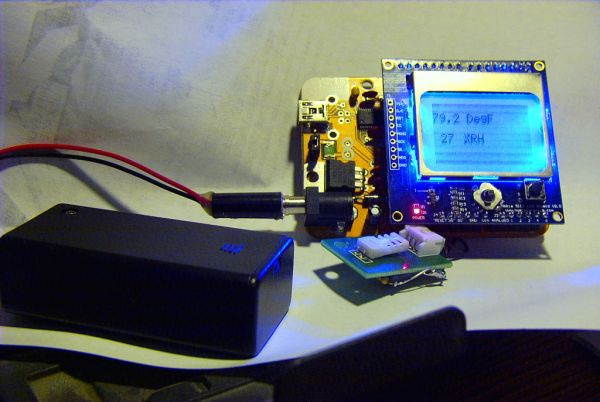# The morse code generator by a PS\2 keyboard using Arduino

Hi, all!
Today I’ m trying to explain you my last project: “The morse code generator by a PS\2 keyboard”

I’ ve used an PS/2 keyboard to send input to my Arduino board (like described in here) and transform it in morse code that activate a speaker and a relay.Components:
– PS\2 keyboard
– Arduino Uno(or others)
– Wires
– 5V relay
– BC547 transistor
– Small speaker
– Recirculation Diode

## Step 1: Setting hardware

The Fritzing project image below can explain almost all, but the hardest thing is connect the keyboard to the Arduino board. As explained here, we must connect the PS\2 connector data pin to the Arduino pin 8, the clock pin to the 2nd, 5V pin to a 5V source and GND pin to GND. If you find a female PS\2 connector you are very lucky and can use it but others can do like me: I’ve use as replacement the metal part, covered by insulating tape, of connectors that are used on the mother boards (for wires of: power and HDD LEDs, power and reset buttons, USB face connectors , ecc. ecc.) .

## Step 2: Software and Conclusions

Here is attached the ‘.ino’ file I’ve made. You’ll find the ‘PS2Keyboard.h’ library in here. Enjoy it./**********
morse.ino
**********/

#include <PS2Keyboard.h>

const int DataPin = 8;
const int IRQpin =  2;

PS2Keyboard keyboard;

void setup() {
delay(1000);
keyboard.begin(DataPin, IRQpin);
}

void loop() {
if (keyboard.available()) {

if (c == ‘a’) {
p();l();
} else if (c == ‘b’) {
l();p();p();p();
} else if (c == ‘c’) {
l();p();l();p();
} else if (c == ‘d’) {
l();p();p();
} else if (c == ‘e’) {
p();
} else if (c == ‘f’) {
p();p();l();p();
} else if (c == ‘g’) {
l();l();p();
} else if (c == ‘h’) {
p();p();p();p();
} else if (c == ‘i’) {
p();p();
} else if (c == ‘j’) {
p();l();l();l();
} else if (c == ‘k’) {
l();p();l();
} else if (c == ‘l’) {
p();l();p();p();
} else if (c == ‘m’) {
l();l();
} else if (c == ‘n’) {
l();p();
} else if (c == ‘o’) {
l();l();l();
} else if (c == ‘p’) {
p();l();l();p();
} else if (c == ‘q’) {
l();l();p();l();
} else if (c == ‘r’) {
p();l();p();
} else if (c == ‘s’) {
p();p();p();
} else if (c == ‘t’) {
l();
} else if (c == ‘u’) {
p();p();l();
} else if (c == ‘v’) {
p();p();p();l();
} else if (c == ‘w’) {
p();l();l();
} else if (c == ‘x’) {
l();p();p();l();
} else if (c == ‘y’) {
l();p();l();l();
} else if (c == ‘z’) {
l();l();p();p();
} else if (c == ‘1’) {
p();l();l();l();l();
} else if (c == ‘2’) {
p();p();l();l();l();
} else if (c == ‘3’) {
p();p();p();l();l();
} else if (c == ‘4’) {
p();p();p();p();l();
} else if (c == ‘5’) {
p();p();p();p();p();
} else if (c == ‘6’) {
l();p();p();p();p();
} else if (c == ‘7’) {
l();l();p();p();p();
} else if (c == ‘8’) {
l();l();l();p();p();
} else if (c == ‘9’) {
l();l();l();l();p();
} else if (c == ‘0’) {
l();l();l();l();l();
} else if (c == ‘ ‘) {
pausa();
} else {
tone(4, 300, 250); //error tone
}
}
}

### Major Components in Project

PS\2 keyboard
– Arduino Uno
– Wires

For more detail: The morse code generator by a PS\2 keyboard using Arduino

#### This Post / Project can also be found using search terms:

• morse code generator by PS2 keyboard using arduino
• arduino morse code
• The Morse Code Generator by a PS/2 Keyboard
• Circuit Details for producing Morse with PS2 Keyboard